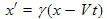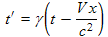There are all sorts of resonances around us, in the world, in our culture, and in our technology. A tidal resonance causes the 55 foot tides in the Bay of Fundy. Mechanical and acoustical resonances and their control are at the center of practically every musical instrument that ever existed. Even our voices and speech are based on controlling the resonances in our throat and mouth. Technology is also a heavy user of resonance. All clocks, radios, televisions, and gps navigating systems use electronic resonators at their very core. Doctors use magnetic resonance imaging or MRI to sense the resonances in atomic nuclei to map the insides of their patients. In spite of the great diversity of resonators, they all share many common properties. In this blog, we will delve into their various aspects. It is hoped that this will serve both the students and professionals who would like to understand more about resonators. I hope all will enjoy the animations.

Origins of Newton's laws of motion

History of mechanical clocks with animations
Understanding a mechanical clock with animations
includes pendulum, balance wheel, and quartz clocks

Saturday, July 9, 2011

Inverting the Lorentz transformsWe need to use inverse Lorentz transforms in several of the derivations in the coming chapters, so we derive these here. The Lorentz transforms from the previous chapter are:(4.1a)(4.1b)(4.1c)(4.1d)

These transforms give the primed or moving coordinates as functions of the unprimed or stationary coordinates. We will invert these to give the stationary coordinates as functions of the moving coordinates.

Inverting y and z transforms:

The y and z coordinates, (4.1b) and (4.1c), are trivial:

y' = y     inverts to give     y = y'     and

z' = z     inverts to give     z = z'    .

Inverting x and t transforms:

The transforms for x and t are intertwined and must be handled together. We repeat the original x and t transforms:

x' = γ (x − Vt)         (4.2a)

t' = γ (t − Vx/c2)      (4.2b)

We solve (4.2a) for x:

x = x'/γ + Vt   (4.3)

and substitute this into (4.2b):.     (4.4)

Multiplying (4.4) by γ yields:

This can be solved for t:

Substituting (4.5) into (4.3) yields:.         (4.6)

The factor in parenthesis in (4.6) can be reduced as follows:.

Using this in (4.6) gives:

SummaryLorentz transforms Inverse Lorentz transforms
x' = γ(x − V t) (4.8a)  x = γ(x' + V t') (4.9a)
y' = y (4.8b) y = y' (4.9b)
z' = z (4.8c)  z = z' (4.9c)(4.8d)(4.9d)

Equations (4.9a) through (4.9d) are the inverse Lorentz transforms. They give the stationary (unprimed) x and t coordinates in terms of the moving coordinates (the primed ones). They are also interesting because except for the minus signs in front of the second terms in each equation, they are identical to the original Lorentz transforms, Equations (4.8a) through (4.8d). They can be had from the original transforms by simply substituting −V in place of V. It means that to the moving reference frame, except for the stationary frame appearing to be moving in the opposite direction, it has the same length contraction and time dilation that people in the stationary frame see when looking at the moving frame.